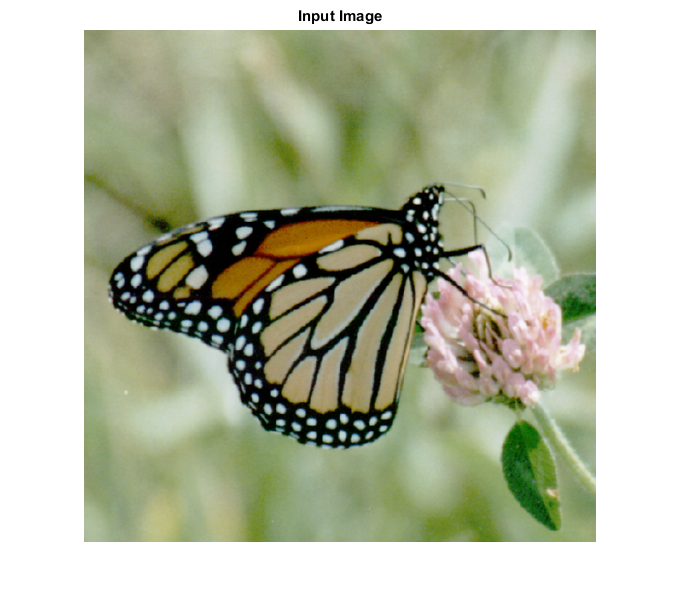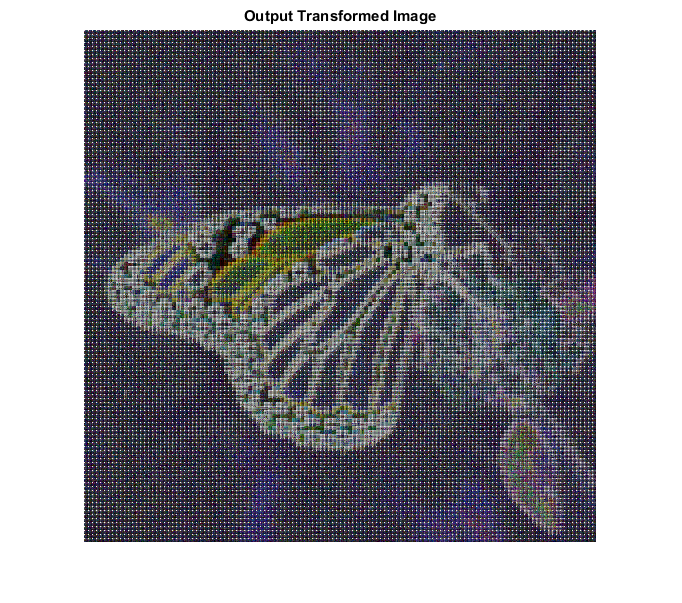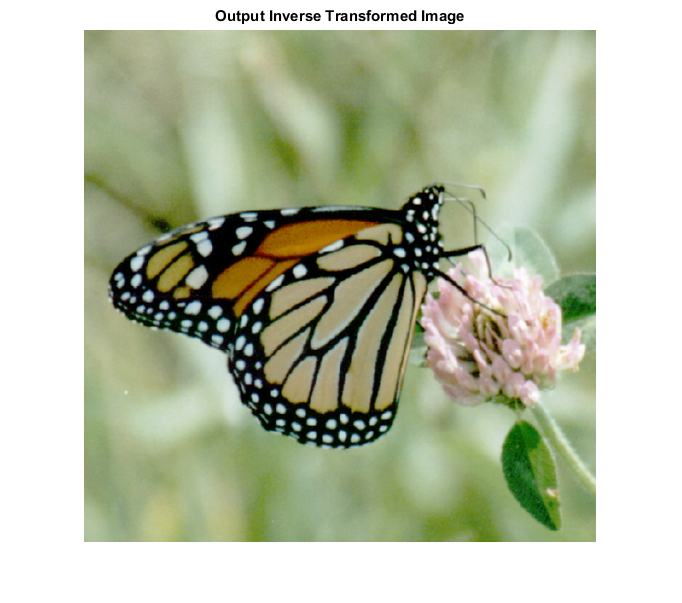## SYNTAX

[ img ] = iwalhad_cvip( input_img, block_size )

Input Parameters Include:

• input_spect - The Walsh/Hadamard spectrum of an image, obtained by walhad_CVIP().
• block_size - Should be a power of 2 or [].if empty,then functions finds the nearest power of 2 and zero pad the image,to that block size.Any other value smaller than image size will partiotion the image to windows of that block size and inverse WHT will be calculated separately in each window.

Output Parameter Include:

• img - The original image with the given spectrum.

## Reference

1. Scott E Umbaugh. DIGITAL IMAGE PROCESSING AND ANALYSIS: Applications with MATLAB and CVIPtools, 3rd Edition.

## Example

```% Read image

% Block size

block_size = 4;

img = remap_cvip(v);

% Display input image

figure;imshow(input_img);title('Input Image');

% Display output image

figure;imshow(remap_cvip(log(1+abs(spect))),[]);title('Output Transformed Image');

figure;imshow(img,[]);title('Output Inverse Transformed Image');
```